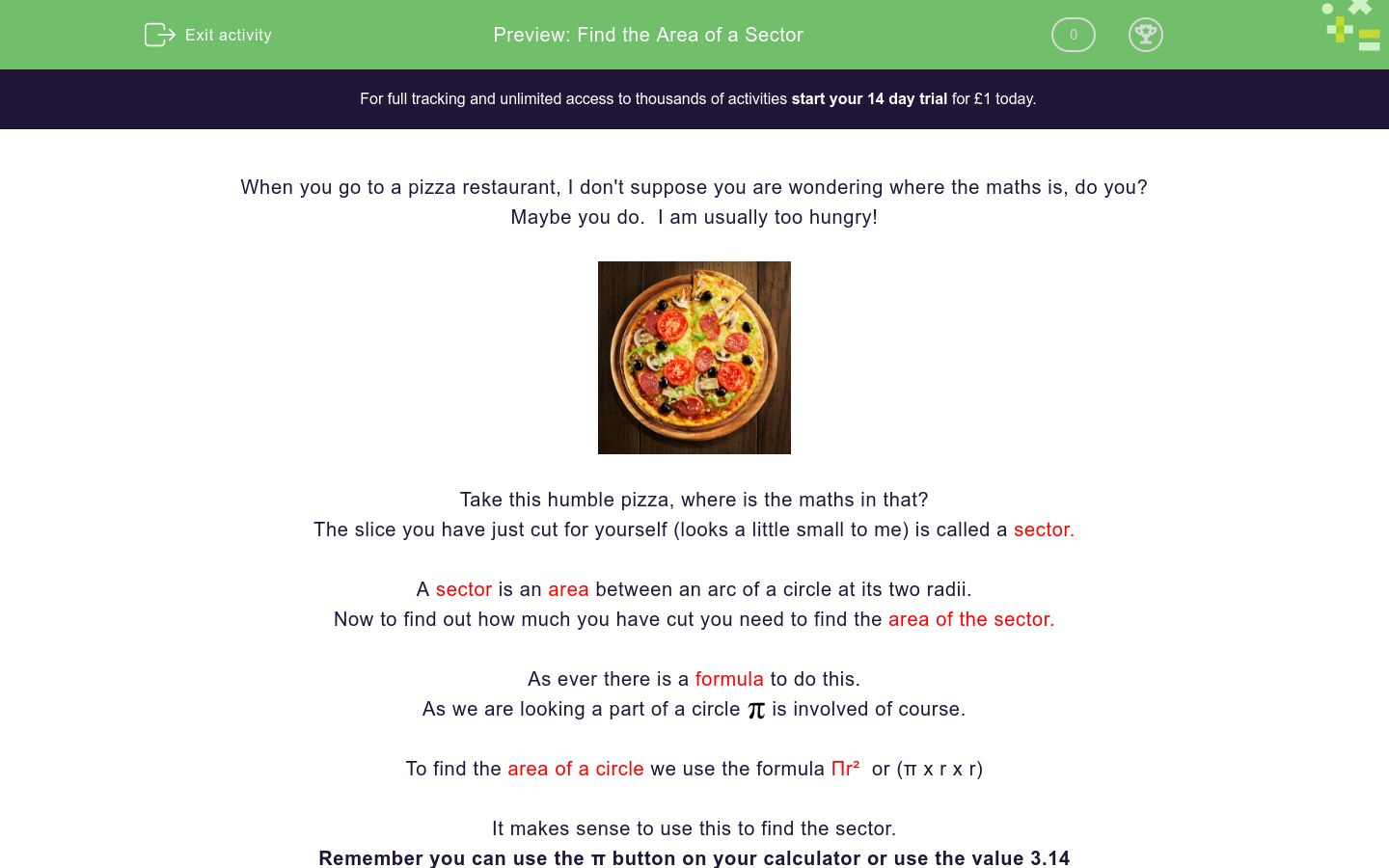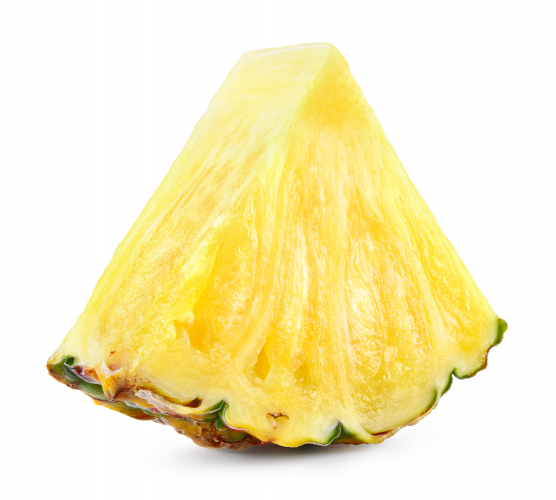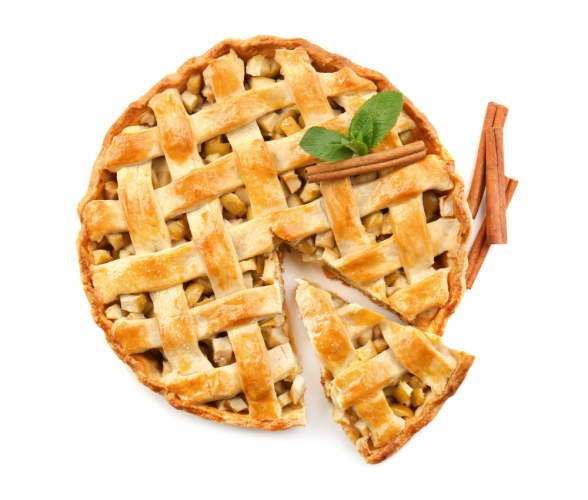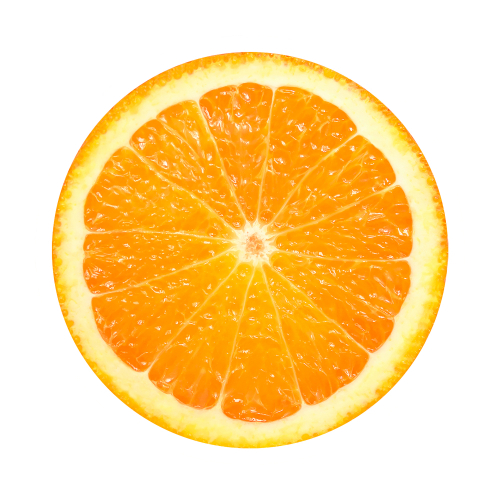# Find the Area of a Sector

In this worksheet, students will learn the formula for finding the area of a sector and be able to apply it.Key stage:  KS 4

GCSE Subjects:   Maths

GCSE Boards:   AQA, Eduqas, Pearson Edexcel, OCR

Curriculum topic:   Geometry and Measures, Mensuration

Curriculum subtopic:   Mensuration and Calculation, Area Calculations

Difficulty level:### QUESTION 1 of 10

When you go to a pizza restaurant, I don't suppose you are wondering where the maths is, do you?

Maybe you do.  I am usually to hungryTake this humble pizza, where is the maths in that?

The slice you have just cut for yourself (looks a little small to me) is called a sector.

A sector is an area between an arc of a circle at its two radii.

Now to find out how much you have cut you need to find the area of the sector.

As ever there is a formula to do this.

As we are looking a part of a circleis involved of course.A small gift is given to us.  To find the area of a circle we use the formula Πr²  or (π x r x r)

It makes sense to use this to find the sector.

Remember you can use the π button on your calculator or use the value 3.14

Area of a sector =  angle/360 x π x radius x radius      (angle/360 x πr²)

Lets calculate how hungry you are.The radius of the pizza is 6 cm and the angle is 50°

50 ÷ 360 x π x 6 x 6 = 15.71 cm²To save a lot of writing down in mid calculation, it is best if you use a scientific calculator as you can put the whole formula in at once.

The calculator is clever enough to know the value of pi too.Find the area of the sector of number 20.

The angle is 18° and the radius is 8.5 cm.Calculate the area of the red sector.

Angle = 30°

Answers have been rounded to 2 decimal places (2 d.p)

13.24 cm²

14.86 cm²

12.83 cm²

11.29 cm²The angle is 63°

Find the sector area between 12 and 2.

13.24 cm²

14.86 cm²

12.83 cm²

11.29 cm²Angle = 78°

Calculate the sector area of this slice of pineapple.

 89.10 cm² 98.01 cm² 90.23 cm² Area of the sectorFind the area of this slice of pie.

Angle = 47°

17.33 cm²

13.77 cm²

12.22 cm²

15.55 cm²Find the area of 4 segments of this orange.  The radius is 5 cm and the angle at the centre is 59°

Round your area to 2 decimal places (2 d.p).Find the area of one of the larger triangular sectors of this car wheel.

Angle is 65° and the radius is 7 cm.Angle = 32°  Radius = 4 cm

 3.87 cm² 4.47 cm² 5.474 cm² The area of this sector isFind the answer of the largest section of this pie chart.

Angle = 130°  radius = 6 cm.

Lets finish with a flourish and match up.

## Column B

Angle of sector 48° radius 6 cm
Area = 13.73 cm²
Angle of sector 52° radius 5.5 cm
Area = 8.66cm²
Angle of sector 81° radius 3.5 cm
Area = 15.08 cm²
Angle of sector 120° radius 4.5 cm
Area = 21.21 cm²
• Question 1Find the area of the sector of number 20.

The angle is 18° and the radius is 8.5 cm.

11.35
EDDIE SAYS
Applying the formula correctly is the key. Having a scientific calculator is really useful here. 18 ÷ 360 x π x 8.5 x 8.5 = 11.35 cm² If only hitting the dartboard was that easy.
• Question 2Calculate the area of the red sector.

Angle = 30°

Answers have been rounded to 2 decimal places (2 d.p)

12.83 cm²
EDDIE SAYS
Quite often we are given written information about sectors. We just need to make sure we extract the correct information to put into our formula. 30 ÷ 360 x π x 7 x 7 = 12.83 cm²
• Question 3The angle is 63°

Find the sector area between 12 and 2.

EDDIE SAYS
Why time is it? Time to work out the sector of course. 63 ÷ 360 x π x 6 x 6 = 11.35 cm² On a cuckoo clock, why didn't the cuckoo come out of the door? He had stage fright...
• Question 4Angle = 78°

Calculate the sector area of this slice of pineapple.

 89.10 cm² 98.01 cm² 90.23 cm² Area of the sector
EDDIE SAYS
Why did the pineapple only get half way to solving this? It ran out of juice. Unlike you of course. This is why learning the formula is so important. 78 ÷ 360 x π x 12 x 12 = 11.35 cm²
• Question 5Find the area of this slice of pie.

Angle = 47°

17.33 cm²
EDDIE SAYS
What is an Australian ghost favourite pie? Boo Meringue. Hopefully the formula isn't scoring you off. It really is a case of just practicing it. 47÷ 360 x π x 6.5 x 6.5 = 17.33cm²
• Question 6Find the area of 4 segments of this orange.  The radius is 5 cm and the angle at the centre is 59°

Round your area to 2 decimal places (2 d.p).

12.87
EDDIE SAYS
Keep going with the formula.. either that or there will be more terrible jokes. 59 ÷ 360 x π x 5 x 5 = 12.87 cm²
• Question 7Find the area of one of the larger triangular sectors of this car wheel.

Angle is 65° and the radius is 7 cm.

EDDIE SAYS
It is amazing where we can see sectors in real life. You never know when you may be asked to calculate one. As long as you have an angle and a radius you are good to go. 65 ÷ 360 x π x 7 x 7 = 127.79 cm²
• Question 8Angle = 32°  Radius = 4 cm

 3.87 cm² 4.47 cm² 5.474 cm² The area of this sector is
EDDIE SAYS
Remember because finding a sector is to do with a circle that π always comes into the calculation. 32 ÷ 360 x π x 4 x 4 = 4.47 cm²
• Question 9Find the answer of the largest section of this pie chart.

Angle = 130°  radius = 6 cm.

40.84
EDDIE SAYS
Isn't maths so much easier when we follow the instructions given to us. They are always there is some kind of formula. Just wish at times there were not so many to learn!! 130 ÷ 360 x π x 6 x 6 = 13.61 cm²
• Question 10

Lets finish with a flourish and match up.

## Column B

Angle of sector 48° radius 6 ...
Area = 15.08 cm²
Angle of sector 52° radius 5....
Area = 13.73 cm²
Angle of sector 81° radius 3....
Area = 8.66cm²
Angle of sector 120° radius 4...
Area = 21.21 cm²
EDDIE SAYS
I hope you are now seeing the formula in your sleep. Angle ÷ 360 x π x r x r Remember to always check how many decimal places you may need to round something to.
---- OR ----

Sign up for a £1 trial so you can track and measure your child's progress on this activity.

### What is EdPlace?

We're your National Curriculum aligned online education content provider helping each child succeed in English, maths and science from year 1 to GCSE. With an EdPlace account you’ll be able to track and measure progress, helping each child achieve their best. We build confidence and attainment by personalising each child’s learning at a level that suits them.

Get started